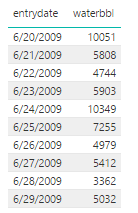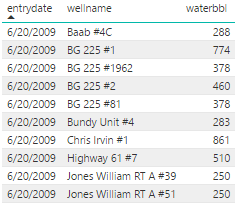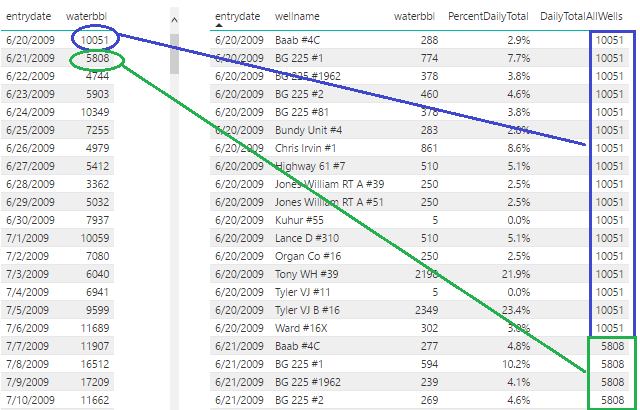# Power BI Tip: Daily Total and Percent of Daily Total DAX Expressions

Here is a quick tip for analyzing daily data in Power BI. If you have a query or dataset that contains a date, a category, and a value measure for that category and you want to create a static measure that always returns the total value for all categories, here is the DAX expression you would use:

`DailyTotalAllCategories = CALCULATE(sum(Query1[value]),ALL(Query1[categoryname]))`

You could then use this daily total DAX measure in calculation of the percentage of the total for each category with this formula:

`PercentDailyTotal = DIVIDE(SUM(Query1[value]),DailyTotalAllCategories)`

If you are only interested in the percent daily total you could bypass the DailyTotalAllCategories measure altogether and your formula would look like this:

```PercentDailyTotal = DIVIDE(
SUM(Query1[value]),
CALCULATE(
sum(Query1[value]),
ALL(Query1[category])
)
)```

Speaking in generalities can be a little hard to follow. A simple oil and gas implementation of these concepts is after the break.

Recently I had some historical data from a client’s previous production system given to me for analysis. The oil and gas looked fine at the well level and company level. The water was a different story.

The water totals at the company level looked consistent, but when individual wells were graphed there would be random spikes and dips. This indicated that there might have been an allocation problem in the legacy system and that water was taken from one well and allocated to another.

I decided I wanted to see an individual well’s daily water total as a percentage of the overall company’s water production total. That’s when I turned to PowerBI.

I created a simple query that gathered all of the water values for all of the wells. It returned 3 columns: date, waterbbl, wellnameIn Power BI if you insert a table and add just entrydate and waterbbl, PowerBI will automatically total the water at the daily level. When you add the well to the report it will show you the well water value at the well level.

Note: The data and screenshots are from randomized values. No actual client data appears belowTo add the daily total and percentage of the individual well to the daily total, the following DAX measures were created

`DailyTotalAllWells = CALCULATE(sum(Query1[waterbbl]),ALL(Query1[wellname]))`
```PercentDailyTotal = DIVIDE(
SUM(Query1[waterbbl]),
CALCULATE(
sum(Query1[waterbbl]),
ALL(Query1[wellname])
)
)```

This is what it looked like when they were added to the tableOf course further analysis was needed to cleanse the data, but I thought I’d pass on a quick tip that could hopefully save someone some time.